# 【muzzik教程】：我所理解的SDF（阴影，描边，外发光...）｜社区征文## # 效果图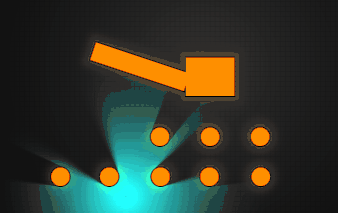## # 前言

SDF 是什么？

SDF 的全称是 Signed Distance Field（有符号距离场），那么有符号是什么？距离场又是什么呢？

有符号：指的是正数和负数，正数代表在物体外，负数代表在物体内

距离场：其中的 数值正是代表到物体表面的距离，0就代表物体表面，例如数值 5 就代表当前点在物体外，距离表面还有 5 的距离，负数则相反

• SDF 渲染
• 相交
• 融合
• 抵消
• 减去
• 描边
• 外发光
• 内发光
• 硬阴影
• 软阴影

## # 什么是 Shader?

Shader 其实是一段GLSL（OpenGL着色语言）程序，而 WebGL 则是为了方便浏览器使用而封装的OpenGL

• 顶点着色器：模型由三角面组成，三角面由顶点组成，而顶点作色器就负责 顶点的坐标控制，可以用来实现布料、水体等等…

• 片段着色器：片段着色器则是负责 渲染位置的颜色输出

• clamp(x, y, z)：x < y 返回 y，x > z 返回 z，否则返回 x

• mix(x, y, z)：x, y 的线性混叠， x(1 - z) + y * z

• length(x)：返回一个向量的模（长度），即 sqrt(dot(x,x))

• sign(x)：x < 0 时返回 -1，x == 0 返回 0，x > 0 返回 1

## # 如何用 SDF 画一个圆？

• 问题1：参数 p 是什么？

• 解答：p 是当前渲染点的位置，因为是 2d，所以只有 xy
• 问题2：参数 r 是什么？

• 解答：r 就是想要绘制的圆半径

“举个栗子”： 圆半径5，圆在 0,0 点（所有公式皆基于 0,0 点），渲染点在 0,3 点，这时 length ( p ) = 3，3 - 5 = -2，则我们离圆的表面有 2 的距离，负数代表渲染点在物体内

### 在片段着色器“画”出来

• output_v4：片段着色器输出的颜色
• float dist_f：距离场
• vec4 color_v4：物体颜色
``````output_v4 = mix(output_v4, color_v4, clamp(-dist_f, 0.0, 1.0));
``````

- dist_f：负负得正，所以在物体内部 clamp 结果就是一个有效值，在物体外部就是负数（clamp结果为0），最终结果就为原本的 output_v4，所以只有在物体内部 mix 才会生效

“深入理解”

## # 旋转，跳跃，我闭着眼~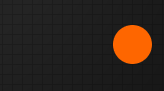### 如何实现？

``````vec2 translate(vec2 render_v2_, vec2 move_v2_) {
return render_v2_ - move_v2_;
}
``````（举栗）

``````float dist_f = sdf_circle(translate(render_v2_, vec2(100.0, 100.0)), 10.0);
``````
• dist_f 便是我们通过 sdf 函数求得平移 vec2(100.0, 100.0) 后的距离场

### 平移实现了，旋转呢？

• 其实也很简单，学习过矩阵的同学应该知道有个旋转矩阵，我们只需要 将向量 * 二维旋转矩阵，那么就会得到旋转后的点
``````// 逆时针旋转
vec2 rotate_ccw(vec2 render_v2_, float radian_f_) {
return render_v2_ * m;
}

// 顺时针旋转
vec2 rotate_cw(vec2 render_v2_, float radian_f_) {
return render_v2_ * m;
}
``````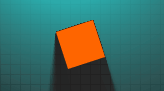## # “正常” 效果### 如何实现？

``````float merge(float dist_f_, float dist2_f_) {
return min(dist_f_, dist2_f_);
}
``````

## # “相交” 效果### 如何实现？

``````float intersect(float dist_f_, float dist2_f_) {
// dist_f_ < 0, dist2_f_ > 0  例 dist_f_ = -2, dist2_f_ = 3，r = 3, 例 dist_f_ = -2, dist2_f_ = 1，r = 1， 则值 > 0
// dist_f_ > 0, dist2_f_ < 0  例 dist_f_ = 2, dist2_f_ = -1，r = 2, 例 dist_f_ = 2, dist2_f_ = -5，r = 2， 则值 > 0
// dist_f_ > 0, dist2_f_ > 0  例 dist_f_ = 1, dist2_f_ = 2，r = 2, 例 dist_f_ = 2, dist2_f_ = 1，r = 2， 则值 > 0
// dist_f_ < 0, dist2_f_ < 0  例 dist_f_ = -2, dist2_f_ = -3，r = -2, 例 dist_f_ = -2, dist2_f_ = -1，r = -1， 则值 < 0
// 所以最终结果只会在 dist_f_ 和 dist2_f_ 重合时展示
return max(dist_f_, dist2_f_);
}
``````

## # “融合” 效果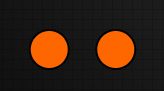### 如何实现？

``````float smooth_merge(float dist_f_, float dist2_f_, float k_f_) {
// k_f_ 如果不超过 abs(dist_f_ - dist2_f_)，那么都是无效值（0 或 1）
float h_f = clamp(0.5 + 0.5 * (dist2_f_ - dist_f_) / k_f_, 0.0, 1.0);
// 假设 k_f_ = 0, dist_f_ = 2, dist2_f_ = 1，则 h_f = 0, mix(...) = dist2_f_, k_f_ * h_f * (1.0 - h_f) = 0，结果为 dist2_f_
// 假设 k_f_ = 0, dist_f_ = 1, dist2_f_ = 2，则 h_f = 1, mix(...) = dist_f_, k_f_ * h_f * (1.0 - h_f) = 0，结果为 dist_f_
// 如果 k_f_  为无效值，那么返回结果将 = min(dist_f_, dist2_f_)，和 merge 结果相同
// 如果 k_f_ 为有效值，那么将返回比 min(dist_f_, dist2_f_) 还要小的值，k_f_  越大，结果越小
return mix(dist2_f_, dist_f_, h_f) - k_f_ * h_f * (1.0 - h_f);
}
``````

## # “抵消” 效果### 如何实现？

``````float merge_exclude(float dist_f_, float dist2_f_) {
// 如果 dist_f_ < 0，dist2_f_ > 0  例 dist_f_ = -2  dist2_f_ = 6, r = -2， 例 dist_f_ = -2  dist2_f_ = 3, r = -2
// 如果 dist_f_ > 0，dist2_f_ < 0  例 dist_f_ = 2  dist2_f_ = -6, r = -6， 例 dist_f_ = -2  dist2_f_ = 3, r = -2
// 如果 dist_f_ > 0，dist2_f_ > 0  例 dist_f_ = 2  dist2_f_ = 6, r = 2， 例 dist_f_ = 5  dist2_f_ = 3, r = 3
// 如果 dist_f_ < 0，dist2_f_ < 0  例 dist_f_ = -2  dist2_f_ = -3, r = 4， 例 dist_f_ = -3  dist2_f_ = -2, r = 4
// 所以最终结果只会将 dist_f_ < 0 && dist2_f_ < 0 的值变成 > 0 的值
return min(max(-dist_f_, dist2_f_), max(-dist2_f_, dist_f_));
}
``````

## # “减去” 效果### 如何实现？

``````float substract(float dist_f_, float dist2_f_) {
// dist_f_ < 0, dist2_f_ > 0  例 dist_f_ = -2, dist2_f_ = 3，r = 3, 例 dist_f_ = -2, dist2_f_ = 1，r = 2， 则值 > 0
// dist_f_ > 0, dist2_f_ < 0  例 dist_f_ = 2, dist2_f_ = -1，r = -1, 例 dist_f_ = 2, dist2_f_ = -5，r = -2， 则值 < 0
// dist_f_ > 0, dist2_f_ > 0  例 dist_f_ = 1, dist2_f_ = 2，r = 2, 例 dist_f_ = 2, dist2_f_ = 1，r = 1， 则值 > 0
// dist_f_ < 0, dist2_f_ < 0  例 dist_f_ = -2, dist2_f_ = -3，r = 4, 例 dist_f_ = -2, dist2_f_ = -1，r = 4， 则值 > 0
// 所以最终结果只会展示 dist2_f_, 且 dist_f_ 和 dist2_f_ 重合时不会展示
return max(-dist_f_, dist2_f_);
}
``````

• dist_f_ > 0 && dist2_f_ < 0 返回 < 0 代表了渲染点不在第一个物体内且在第二个物体内才展示
• 而 dist_f_ > 0, dist2_f_ < 0 返回 > 0 就代表了渲染点同时在两个物体内，也就是抵消效果

## # 如何实现物体描边？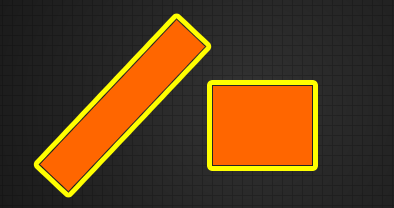• output_v4：片段着色器输出的颜色
• float dist_f：距离场
• vec4 color_v4：描边颜色
• float width_f：描边宽度
``````output_v4 = mix(output_v4, color_v4, abs(clamp(dist_f - width_f, 0.0, 1.0) - clamp(dist_f, 0.0, 1.0)));
``````

## # 如何打造金闪闪（外发光）？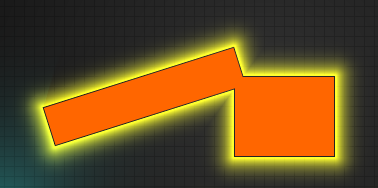• float dist_f：距离场
• vec4 color_v4_：渲染点的颜色
• vec4 input_color_v4_：外发光颜色
``````vec4 outer_glow(float dist_f_, vec4 color_v4_, vec4 input_color_v4_, float radius_f_) {
// dist_f_ > radius_f_ 结果为 0
// dist_f_ < 0 结果为 1
// dist_f_ > 0 && dist_f_ < radius_f_ 则 dist_f_ 越大 a_f 越小，范围 0 ~ 1
float a_f = abs(clamp(dist_f_ / radius_f_, 0.0, 1.0) - 1.0);
// pow：平滑 a_f
// max and min：防止在物体内部渲染
float b_f = min(max(0.0, dist_f_), pow(a_f, 5.0));
return color_v4_ + input_color_v4_ * b_f;
}
``````

dist_f_ 的有效值范围是 （ 0 ~ radius ）

• 如果 dist_f_ > radius_f_
• a_f = 0
• b_f = min(max(0.0, dist_f_), 0) = 0
• 返回值就为 color_v4_，此时为无效值
• 如果 dist_f_ < 0
• a_f = 1
• b_f = min(max(0.0, dist_f_), 1) = 0
• 返回值就为 color_v4_，此时为无效值

## # 内什么啊？内发光啊，什么发光啊？内发光阿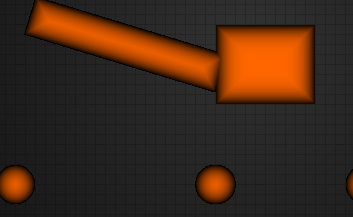（此函数根据上面的外发光改写）

``````vec4 inner_glow(float dist_f_, vec4 color_v4_, vec4 input_color_v4_, float radius_f_) {
// (dist_f_ + radius_f_) > radius_f_ 结果为1
// (dist_f_ + radius_f_) < 0 结果为0
// (dist_f_ + radius_f_) > 0 && (dist_f_ + radius_f_) < radius_f_ 则 dist_f_ 越大 a_f 越大，范围 0 ~ 1
float a_f = clamp((dist_f_ + radius_f_) / radius_f_, 0.0, 1.0);
// pow：平滑 a_f
// 1.0+：在物体内渲染
// max(1.0, sign(dist_f_) * -：dist_f_ < 0 时返回 -1，dist_f_ == 0 返回 0，dist_f_ > 0 返回 1，所以有效值只在物体内部
float b_f = 1.0 - max(1.0, sign(dist_f_) * -(1.0 + pow(a_f, 5.0)));
return color_v4_ + input_color_v4_ * b_f;
}
``````
• 如果 (dist_f_ + radius_f_) > radius_f_
• a_f = 1.0;
• b_f = 1.0 - max(1.0, -2.0) = 0;
• 返回值就为 color_v4_，此时为无效值
• 如果 (dist_f_ + radius_f_) < 0
• a_f = 0.0;
• b_f = 1.0 - max(1.0, 1.0) = 0;
• 返回值就为 color_v4_，此时为无效值

## # 比较硬的阴影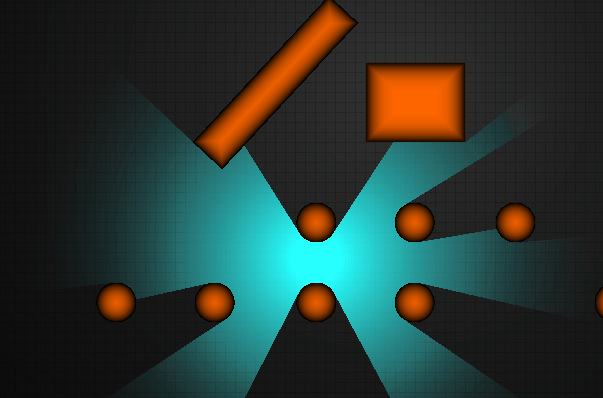（残留像素为录制软件造成）

### 小二，上一份代码，不要辣

• vec2 render_v2_ 渲染点
• vec2 light_v2_ 光源点
``````float shadow(vec2 render_v2_, vec2 light_v2_) {
// 当前渲染位置到光源位置的方向向量
vec2 render_to_light_dir_v2 = normalize(light_v2_ - render_v2_);
// 渲染位置至光源位置距离
float render_to_light_dist_f = length(render_v2_ - light_v2_);
// 行走距离
float travel_dist_f = 0.01;

for (int k_i = 0; k_i < max_shadow_step; ++k_i) {
// 渲染点到场景的距离
float dist_f = scene_dist(render_v2_ + render_to_light_dir_v2 * travel_dist_f);
// 小于0表示在物体内部
if (dist_f < 0.0) {
return 0.0;
}
// abs：避免往回走
// max 避免渲染点距离物理表面过近导致极小耗尽遍历次数，所以有可能会跳过物体距离小于1.0的阴影绘制
travel_dist_f += max(1.0, abs(dist_f));
// travel_dist_f += abs(dist_f); 精确的阴影

// 渲染点的距离超过光源点
if (travel_dist_f > render_to_light_dist_f) {
return 1.0;
}
}
return 0.0;
}
``````

## # 比较软的阴影### 实现方式：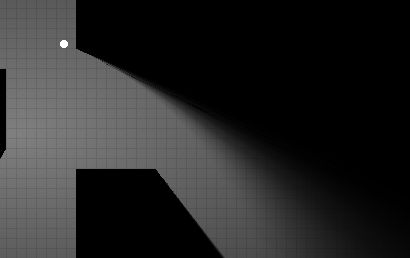### 先上代码

``````float shadow(vec2 render_v2_, vec2 light_v2_, float hard_f_) {
// 当前渲染位置到光源位置的方向向量
vec2 render_to_light_dir_v2 = normalize(light_v2_ - render_v2_);
// 渲染位置至光源位置距离
float render_to_light_dist_f = length(render_v2_ - light_v2_);
// 可见光的一部分，从一个半径开始（最后添加下半部分）；
float brightness_f = hard_f_ * render_to_light_dist_f;
// 行走距离
float travel_dist_f = 0.01;

for (int k_i = 0; k_i < max_shadow_step; ++k_i) {
// 当前位置到场景的距离
float dist_f = scene_dist(render_v2_ + render_to_light_dir_v2 * travel_dist_f);

// 渲染点在物体内部
if (dist_f < -hard_f_) {
return 0.0;
}

// dist_f 不变，brightness_f 越小，在越靠近光源和物体时 brightness_f 越小
brightness_f = min(brightness_f, dist_f / travel_dist_f);

// max 避免渲染点距离物理表面过近导致极小耗尽遍历次数，所以有可能会跳过物体距离小于1.0的阴影绘制
// abs 避免朝回走
travel_dist_f += max(1.0, abs(dist_f));

// 渲染点的距离超过光源点
if (travel_dist_f > render_to_light_dist_f) {
break;
}
}

// brightness_f * render_to_light_dist_f 根据距离平滑, 离光源越近越小，消除波纹线
// 放大阴影，hard_f 越大结果越小则阴影越大, hard_f_ / (2.0 * hard_f_) 使结果趋近于0.5，用于平滑过渡
brightness_f = clamp((brightness_f * render_to_light_dist_f + hard_f_) / (2.0 * hard_f_), 0.0, 1.0);
brightness_f = smoothstep(0.0, 1.0, brightness_f);
return brightness_f;
}
``````

## # 结语

### 吐槽

• WebGL1 是真的很难用，移植期间遇到各种问题，特别是多边形SDF图形，放在webgl1用循环实现性能开销太大，已经放弃多边形

• 引擎的 webgl2 用不了，即使你勾选了webgl2 ，但是编译后代码头还是 #version 100（黑人问号？）

• webgl1性能： 移植前在 shadertoy 占用40%GPU，移植到 creator 占用 90%+GPU，包括官方的 shadowMap

• 明显感觉论坛人气不如之前，提几个问题一个没人回答

43赞

6666给大佬跪了正好了解下

mmmmark

1赞

markmakr